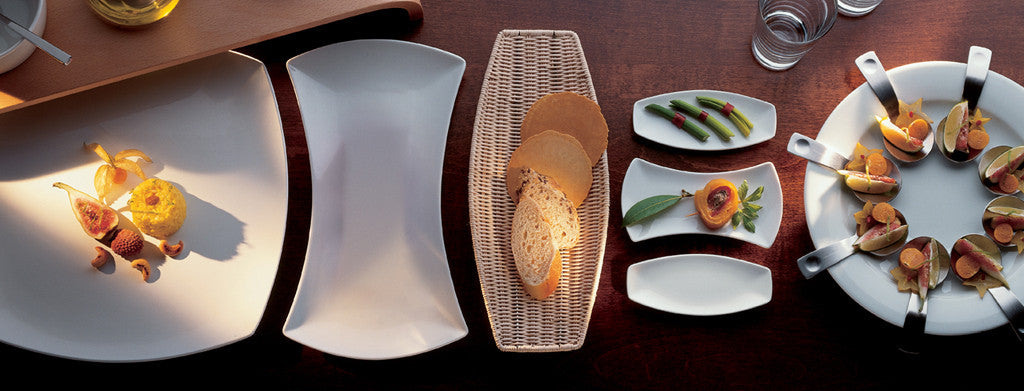Shopping Cart

### Refine

View all

#### Price# Crockery£4.69 each (ex VAT)£2.74 each (ex VAT)£3.25 each (ex VAT)£3.54 each (ex VAT)£4.83 each (ex VAT)£5.93 each (ex VAT)£4.66 each (ex VAT)£22.69 each (ex VAT)£3.83 each (ex VAT)£3.23 each (ex VAT)£4.34 each (ex VAT)£3.47 each (ex VAT)£3.39 each (ex VAT)£6.15 each (ex VAT)£9.66 each (ex VAT)£4.27 each (ex VAT)£2.99 each (ex VAT)£2.07 each (ex VAT)£2.96 each (ex VAT)£5.54 each (ex VAT)£3.14 each (ex VAT)£19.56 each (ex VAT)£3.25 each (ex VAT)£3.25 each (ex VAT)£4.66 each (ex VAT)£4.66 each (ex VAT)£4.34 each (ex VAT)£3.12 each (ex VAT)£2.81 each (ex VAT)£3.21 each (ex VAT)£4.22 each (ex VAT)£2.42 each (ex VAT)£3.50 each (ex VAT)£3.88 each (ex VAT)£28.68 each (ex VAT)£4.32 each (ex VAT)£20.13 each (ex VAT)£5.11 each (ex VAT)£3.57 each (ex VAT)£15.28 each (ex VAT)£2.53 each (ex VAT)£2.83 each (ex VAT)£4.19 each (ex VAT)£4.99 each (ex VAT)£4.99 each (ex VAT)£6.62 each (ex VAT)£6.62 each (ex VAT)£9.38 each (ex VAT)